#Function Repository Resource:

# RoundedPercentageShare

Calculate rounded percentages that always sum to 100%

Contributed by: Jon McLoone
 ResourceFunction["RoundedPercentageShare"][data] generates a list corresponding to the percentage share of the total that each item contributes, ensuring that the result always adds up to exactly 1.

## Examples

### Basic Examples

If you simply calculate percentages and round them, values do not always add up to 1. In this case the total is 0.99:

 In:=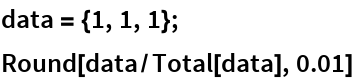Out=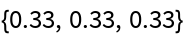In:=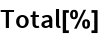Out=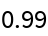RoundedPercentageShare always sums to 1 by allocating spare percentages to the largest contributor:

 In:=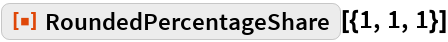Out=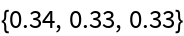### Scope

A second argument controls the amount of rounding:

 In:=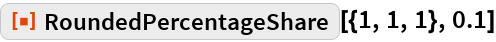Out=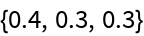In:=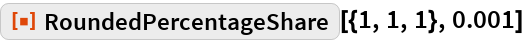Out=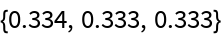## Requirements

Wolfram Language 11.3 (March 2018) or above